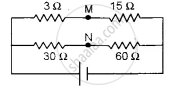# How Much Resistance Should Be Connected to 15 ω Resistor Shown in the Circuit in Figure Below So that the Points M and N Are at the Same Potential: - Physics (Theory)

Sum

How much resistance should be connected to 15 Ω resistor shown in the circuit in figure below so that the points M and N are at the same potential:#### Solution

Let X be the resistance in place of 15 Ω for the points M and N to be at the same potential, then by the condition of balanced wheatstone bridge

"P"/"Q" = "R"/"S"

3/30 = "X"/60

∴ X = ( 3 xx 60 )/30 = 6 Ω

To make the effective resistance of 6 Ω, let R be a resistance connected in parallel with 15Ω resistance, then1/6 = 1/"R" + 1/15

1/"R" = 1/6 - 1/15 = ( 5 - 2 )/30 = 3/30

R = 10 Ω

Concept: Combination of Resistors - Series and Parallel
Is there an error in this question or solution?